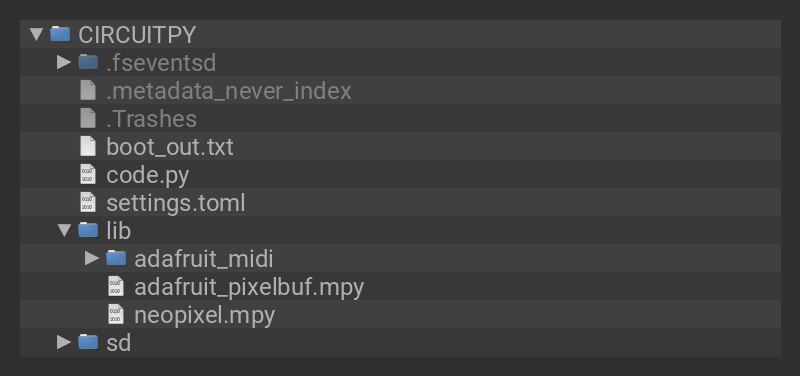You can use your Slider Trinkey as a MIDI CC cross fader! The CircuitPython `analogio` module and the Adafruit CircuitPython MIDI library allow you to use the Slider Trinkey to send MIDI CC commands.

## Code

Click the Download Project Bundle button below to download the necessary libraries and the code.py file in a zip file. Extract the contents of the zip file, and copy the entire lib folder and the code.py file to your CIRCUITPY drive.

Your CIRCUITPY drive should now look similar to the following image:```# SPDX-FileCopyrightText: 2021 Kattni Rembor for Adafruit Industries
#

import time
import board
import analogio
import usb_midi
import neopixel

pixels = neopixel.NeoPixel(board.NEOPIXEL, 2, brightness=1)

position = slider.value  # ranges from 0-65535

midi_in=usb_midi.ports, in_channel=0, midi_out=usb_midi.ports, out_channel=0
)

last_cc_val = 0

# ------- Hue/Sat/Val function to convert to RGB  ------ #
def hsv2rgb(h, s, v):
"""
Convert H,S,V in 0-255,0-255,0-255 format
to R,G,B in 0-255,0-255,0-255 format
Converts an integer HSV (value range from 0 to 255) to an RGB tuple
"""
if s == 0:
return v, v, v
h = h/255
s = s/255
v = v/255
i = int(h * 6.0)
f = (h * 6.0) - i
p = v * (1.0 - s)
q = v * (1.0 - s * f)
t = v * (1.0 - s * (1.0 - f))
i = i % 6
if i == 0:
r, g, b = v, t, p
if i == 1:
r, g, b = q, v, p
if i == 2:
r, g, b = p, v, t
if i == 3:
r, g, b = p, q, v
if i == 4:
r, g, b = t, p, v
if i == 5:
r, g, b = v, p, q
return int(r * 255), int(g * 255), int(b * 255)

hue_a = 0
sat_a = 255
val_a = 255
color_a = hsv2rgb(hue_a, sat_a, val_a)

hue_b = 127
sat_b = 255
val_b = 255
color_b = hsv2rgb(hue_b, sat_b, val_b)

pixels = color_a
pixels = color_b

while True:
cc_val = slider.value // 512  # make 0-127 range for MIDI CC

if abs(cc_val - last_cc_val) > 2:
print(cc_val)
last_cc_val = cc_val
mod_wheel = ControlChange(1, cc_val)
midi.send(mod_wheel)
color_a = hsv2rgb(cc_val, sat_a, val_a)
pixels = color_a
color_b = hsv2rgb(cc_val, sat_b, val_b)
pixels = color_b
time.sleep(0.001)
```

## Usage

Check out the video above for a demo and code explainer!

You can use the code to control any MIDI CC parameter you like in pretty much any synth or DJ software, as well as any hardware synths that can act as a USB host.

If necessary, you can also adjust the MIDI channel and CC number in code. By default this uses MIDI channel 1 and CC number 1 -- a.k.a, Mod Wheel.

This guide was first published on Jun 16, 2021. It was last updated on Dec 10, 2023.